### Home > CALC > Chapter 1 > Lesson 1.4.1 > Problem1-140

1-140.
1. Given the function f(x) sketched below, Homework Help ✎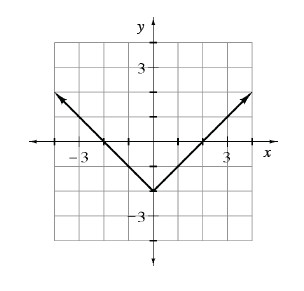1. Sketch f(−x).

2. Sketch −f(x).

3. Sketch f(f(x)).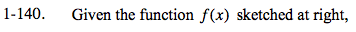Start by making a table of values for the given function.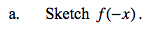Give each of the x-values in your table the opposite sign and graph the new function.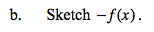Since f(x) = y, give each of the y-values in your original table the opposite sign. Then graph the new function.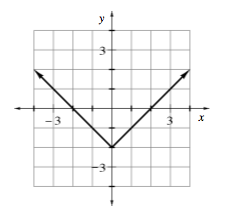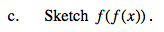Add a third column to your original table labeled f(f(x)). Then, for example, f(f(–3)) = f(1) = –1.